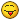Name E-mail Your e-mail address will never be displayed on the site. Subject Message [quote="Aishah Ismail" post=7304]pls advice where you gotten the 105 formula qns? I would like to practice. kindly email me bteismaa@hotmail.com[/quote]

Topic History of : PM Formulas

Max. showing the last 6 posts - (Last post first)
3 years 5 months ago #7307

Yasir MehmoodHi Aishah,

You can purchase the formulas and the 105 sample questions here.
https://www.project-management-prepcast.com/pmp-exam/the-pm-formulas
3 years 5 months ago #7304

Aishah Ismailpls advice where you gotten the 105 formula qns?
I would like to practice. kindly email me This email address is being protected from spambots. You need JavaScript enabled to view it.
3 years 5 months ago #7297

Yasir MehmoodHi Damien,

I didn't have the question so I took the long route but basically if you use CPI with more decimal places the answer works out. As you noticed rounding the CPI up even one decimal place makes a fairly large difference in the final answer.

You mentioned CPI = 240,000 / 253.700 so that tells me the question gives you the EV as 240,000 and AC as 253,700
You mentioned EAC = 700,000 / CPI which tells me that the question gives you BAC as 700,000
(I now know I didn't need to do that)

CPI = 240,000 / 253,700 = 0.945945945
EAC = BAC / CPI => 700,000 / 0.945945945 => 740,000.00074
ETC = EAC - AC => 740,000.00074 - 253,700 => 486,300.00074 => 486,300
3 years 5 months ago #7295

DamienHi
I purchased the 105 Formula Questions.
I'm looking to clarify the answer to question No.73. The answer given is A) 486,300. When I calculate using the correct formulas I get an answer of CPI = 240,000 / 253,700 = 0.9459. Is this ratio that added to the nearest decimal point, i.e. 0.95 ??
Using the answers ratio of 0.946 EAC = 700,000 / 0.946 = 739,957.716
• ETC = EAC – AC = 739,958 – 253,700 = 486,258
If you round off 0.946 to 0.95 you get EAC = 700,000 / 0.95 = 736,842,10
• ETC = EAC – AC = 736,842 – 253,700 = 483,142

Regards
Damien

OSP INTERNATIONAL LLC
OSP INTERNATIONAL LLC
Training for Project Management Professional (PMP)®, PMI Agile Certified Practitioner (PMI-ACP)®, and Certified Associate in Project Management (CAPM)®